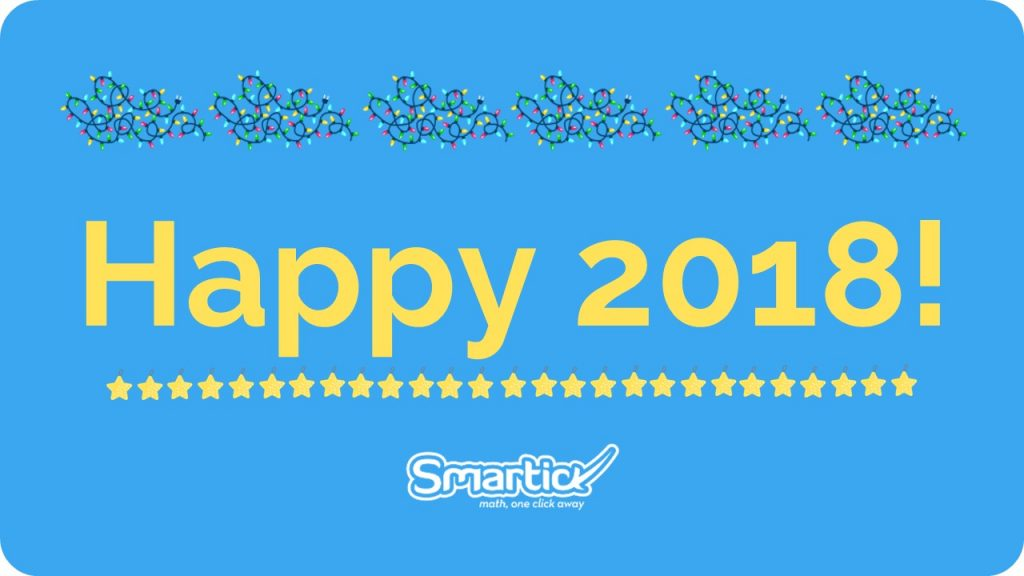Smartick is an advanced online program that teaches kids math and coding in only 15 min. a day

Jul09

# Practising Roman Numerals

In today’s post, we’re going to do some exercises to help practise Roman numerals. Let’s go!

If you want to review them before we start, take a look at one of our previous posts where we explain them in detail.

#### The Current Year in Roman Numerals

To start with, we’re going to do a very simple exercise: write the current year, 2018, in Roman numerals.First, we’ll break down 2018 into each of the numbers that we can represent with Roman numerals:

2018 = 1000 + 1000 + 10 + 5 + 1 + 1 + 1

The next step is easy, all we have to do is substitute these numbers for the corresponding letters and put them in order, making sure we place them in order of value from highest to lowest (since they add together).

We replace each number with the letter that represents it:

M -> 1.000

X -> 10

V -> 5

I -> 1

And now all we have to do is arrange them, as we said, in order of value from highest to lowest:

2018 = 1000 + 1000 + 10 + 5 + 1 + 1 + 1 -> M M X V I I I

#### Your Birth Year in Roman Numerals

Now you can try writing the year you were born in Roman numerals!

If, for example, you were born in the year 2009, it’ll be a bit more complicated to write it in Roman numerals, because there are some numbers that are formed by subtracting.

But we’ll do it step by step so you can see how it’s done.

As you know, changing the year 2000 is really easy, but as you’ll see, we have to be a bit more careful with the 9.

If we represent it using the same additive method as before, we’ll get VIIII. But we can’t put four I’s together, so we’ll have to use subtraction. In other words, we have to take 9 as:

9 = 10 – 1

Remember that when we subtract Roman numerals, we put the number we’re subtracting in front of (one position to the left of) the number we want to subtract it from. So since 10 is represented by X and 1 is represented by I we get:

9 = 10 – 1 -> I X

Now we can represent 2009!

2009 = 1000 + 1000 + (10 – 1) -> M M I X

#### Work out the year on the Puerta de Alcalá in Madrid, Spain

Now we’re going to find out what year is written on the Puerta de Alcalá in Madrid: MDCCLXXVIII.

If you’ve ever been to this city, you might have seen it for yourself!To convert this Roman numeral, all you have to do is substitute each one of the letters for the number it represents and add them, like this:

MDCCLXXVIII -> 1000 + 500 + 100 + 100 + 50 + 10 + 10 + 5 + 1 + 1 + 1 = 1778

And that’s it! It has these Roman numerals on it because it was inaugurated in the year 1778.

Why don’t you go out and find the Roman numerals written on the monuments in your city?

It’s a fun idea to take a walk, take some photos of all the ones you find, and then work out the numbers they’re hiding.

What did you think of this post? Did it help you practise Roman numerals? Don’t forget to share it so that others can practise too!